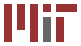## 6.046: Introduction to Algorithms

### Massachusetts Institute of Technology - Spring 2004

#### Course Objectives

This course introduces students to the analysis and design of computer algorithms. Upon completion of this course, students will be able to do the following:

• Analyze the asymptotic performance of algorithms.
• Demonstrate a familiarity with major algorithms and data structures.
• Apply important algorithmic design paradigms and methods of analysis.
• Synthesize efficient algorithms in common engineering design situations.

#### Course Outcomes

Students who complete the course will have demonstrated the ability to do the following:

• Argue the correctness of algorithms using inductive proofs and loop invariants.
• Analyze worst-case running times of algorithms using asymptotic analysis. Compare the asymptotic behaviors of functions obtained by elementary composition of polynomials, exponentials, and logarithmic functions. Describe the relative merits of worst-, average-, and best-case analysis.
• Analyze average-case running times of algorithms whose running time is probabilistic. Employ indicator random variables and linearity of expectation to perform the analyses. Recite analyses of algorithms that employ this method of analysis.
• Explain the basic properties of randomized algorithms and methods for analyzing them. Recite algorithms that employ randomization. Explain the difference between a randomized algorithm and an algorithm with probabilistic inputs.
• Analyze algorithms using amortized analysis, when appropriate. Recite analyses of simple algorithms that employ this method of analysis. Describe different strategies for amortized analysis, including the accounting method and the potential method.
• Describe the divide-and-conquer paradigm and explain when an algorithmic design situation calls for it. Recite algorithms that employ this paradigm. Synthesize divide-and-conquer algorithms. Derive and solve recurrences describing the performance of divide-and-conquer algorithms.
• Describe the dynamic-programming paradigm and explain when an algorithmic design situation calls for it. Recite algorithms that employ this paradigm. Synthesize dynamic-programming algorithms and analyze them.
• Describe the greedy paradigm and explain when an algorithmic design situation calls for it. Recite algorithms that employ this paradigm. Synthesize greedy algorithms and analyze them.
• Explain the major algorithms for sorting. Recite the analyses of these algorithms and the design strategies that the algorithms embody. Synthesize algorithms that employ sorting as a subprocedure. Derive lower bounds on the running time of comparison-sorting algorithms, and explain how these bounds can be overcome.
• Explain the major elementary data structures for implementing dynamic sets and the analyses of operations performed on them. Recite algorithms that employ data structures and how their performance depends on the choice of data structure. Synthesize new data structures by augmenting existing data structures. Synthesize algorithms that employ data structures as key components.
• Explain the major graph algorithms and their analyses. Employ graphs to model engineering problems, when appropriate. Synthesize new graph algorithms and algorithms that employ graph computations as key components, and analyze them.
• Demonstrate a familiarity with applied algorithmic settings --- such as computational geometry, operations research, security and cryptography, parallel and distributed computing, operating systems, and computer architecture --- by reciting several algorithms of importance to different fields.